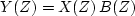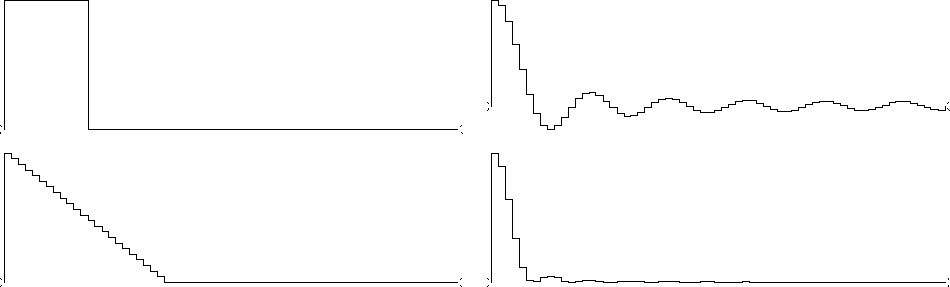Next: SETTING UP THE FAST Up: SYMMETRIES Previous: Plot interpretation

## Convolution in the frequency domain

Let.The coefficients yt can be found from the coefficients xt and bt by convolution in the time domain or by multiplication in the frequency domain. For the latter, we would evaluate both X(Z) and B(Z) at uniform locations around the unit circle, i.e., compute Fourier sums Xk and Bk from xt and bt. Then we would form Ck=Xk Bk for all k, and inverse Fourier transform to yt. The values yt come out the same as by the time-domain convolution method, roughly that of our calculation precision (typically four-byte arithmetic or about one part in 10-6). The only way in which you need to be cautious is to use zero padding greater than the combined lengths of xt and bt.

An example is shown in Figure 8. It is the result of a Fourier-domain computation which shows that the convolution of a rectangle function with itself gives a triangle. Notice that the triangle is clean--there are no unexpected end effects.

 box2triangle Figure 7 Top shows a rectangle transformed to a sinc. Bottom shows the sinc squared, back transformed to a triangle.Because of the fast method of Fourier transform described next, the frequency-domain calculation is quicker when both X(Z) and B(Z) have more than roughly 20 coefficients. If either X(Z) or B(Z) has less than roughly 20 coefficients, then the time-domain calculation is quicker.Next: SETTING UP THE FAST Up: SYMMETRIES Previous: Plot interpretation
Stanford Exploration Project
10/21/1998